|

# 松下“适悦光”三大技术创新 打造智能家居生态

在经济持续发展的驱动下，我国家居市场消费水平不断升级，单纯的价格优势已不再是消费者选择商品的唯一决定因素，人们更加注重产品功能体验之上的附加价值。中国的家居市场呈现出全新的面貌，智能化的产品更加受到消费者喜爱，依靠一台智能手机远程操控家居环境已成为未来家居生活的趋势之一。

与此同时，用户对于商品的品质和服务体验需求也在日益升级，对于当下的家居家装用户而言，品质化、个性化、智能化、健康化等都已成为商品选购的决定要素。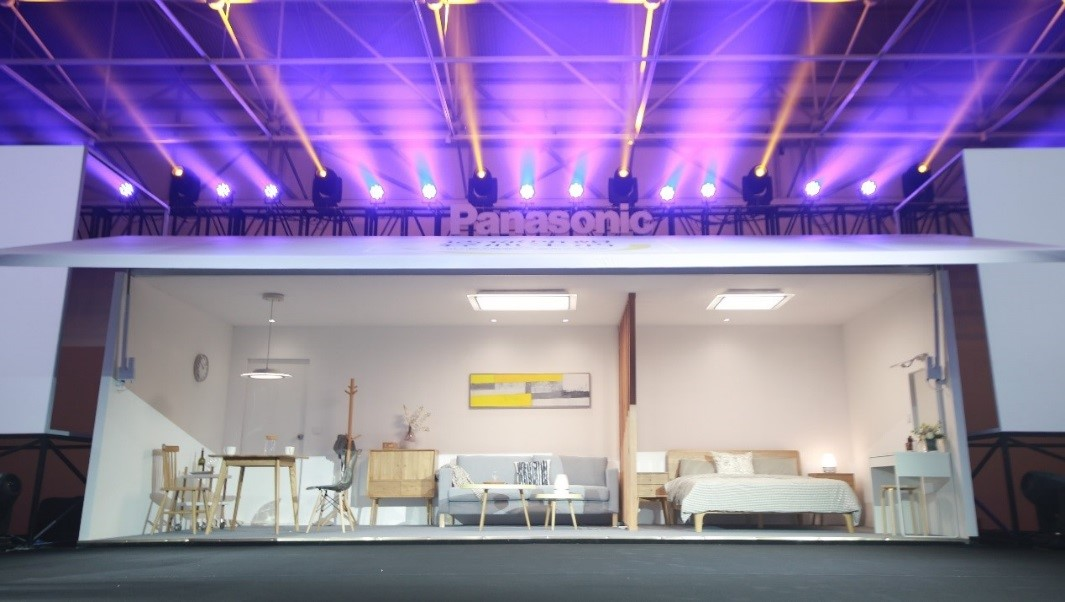松下电器（中国）有限公司 环境方案公司近期推出的全新照明旗舰产品——“适悦光”正是根据目前消费者多样化的需求而量身打造。该系列产品从家庭中不同居住者的角度出发，为家居生活设计和提供最佳的照明解决方案，引领家居生活向松下照明所憧憬的 “健康“、”舒适 “、“品位”的生活空间而进发。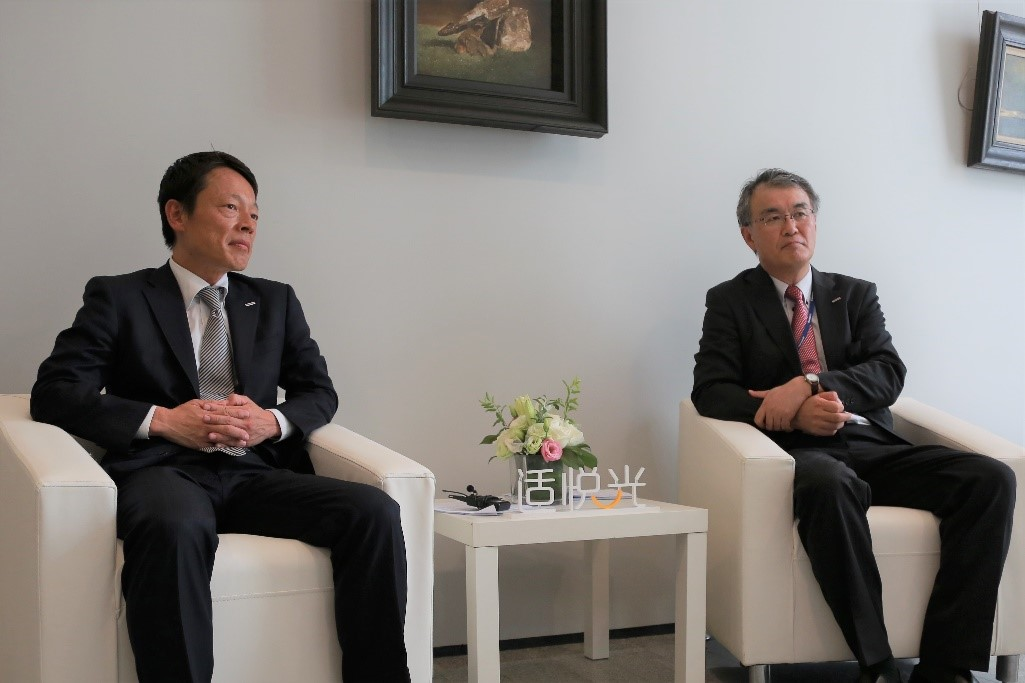松下电器(中国)有限公司 环境方案公司少路政彦总经理与松下电气机器（北京）有限公司山中直总经理接受搜狐焦点专访

三大创新技术引领场景化智能照明时代

“单纯的提供产品已不能满足消费者对智能家居的消费需求。松下照明深刻洞察这一市场现状，从单品生产者成功晋升为整体照明解决方案的提供者。”松下电器（中国）有限公司 环境方案公司总经理

少路政彦表示，“适悦光”系列产品的推出，正是松下照明场景化、智能化探索中的重要一步。

本次上市的“适悦光”新品具有适景蓝牙操控系统、适悦调色以及美光色三大技术优势，而这些优势背后则是松下照明不断努力的技术创新。

适景蓝牙操控系统——

住宅智能化正成为目前的家居发展的主流趋势之一，“适悦光”搭载适景蓝牙操控系统，可以实现多盏照明灯具的整体操控，可根据不同时间和场景的需要实现多种情景设置，一方面模仿一天中不同时段的自然光，另一方面根据不同使用者所进行的室内活动设计最适合的光环境，无论学习、用餐、欣赏电影、接待客人，均可带给使用者舒适而愉悦的照明体验。

适悦调色——

松下照明在设计“适悦调色系列“时，运用了荷兰物理学家A.A.Kruithof研究发现的“克鲁托夫舒适型曲线”，在改变灯光亮度的同时实现光色的均衡变化，排除了让人产生不悦的亮度和色温组合。无论灯光如何变化，都能让人一直处在舒适而健康的光环境中。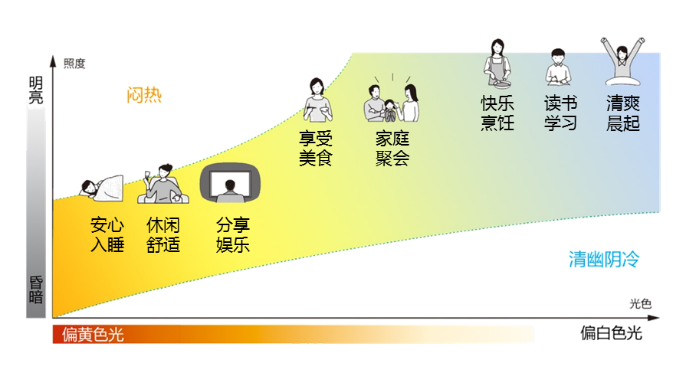美光色——

美光色功能主要用于镜前灯。由于人工光线与自然光线的差异，在人工光线的照射下人们在镜子中看到的自己可能与实际情况存在很大的色差。美光色功能是通过对光线的波长进行控制，让灯光更大程度的接近自然光，使人的肤色更显鲜活、亮丽，让使用者在镜前看到最真实、最美丽的自己。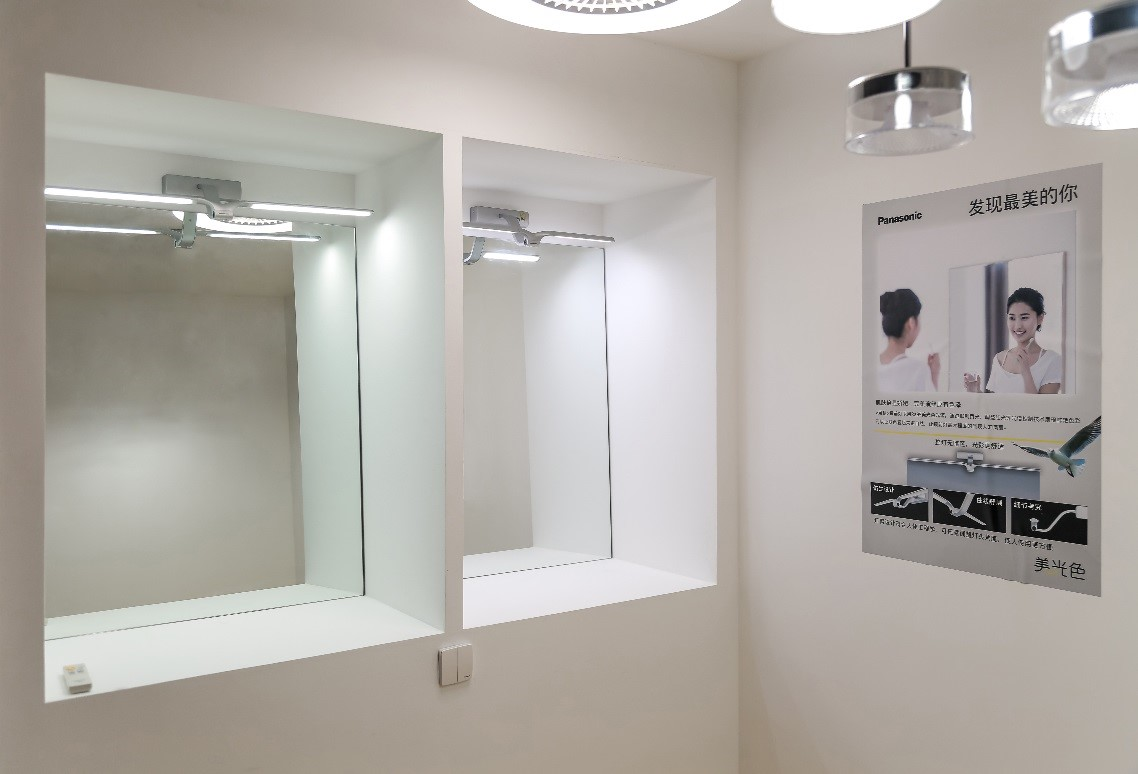“美光色”产品展示

“适悦光”系列的三大技术创新，基于松下照明长期以来对照明技术的深入研究以及对消费趋势的超前探索，在照明产品智能化的趋势方兴未艾之时，松下照明已走在了场景化照明时代的前列。

“健康”、“余暇”、“品味”的高质感生活提案

一直以来，松下照明以实现高品质的制造在市场上获得了高度评价。但松下照明的愿景并不止于此。“我们发现仅仅将日本的技术照搬到中国是不够的，还要开发出符合中国人使用习惯的产品，才能够在中国市场获得更长远的发展。”松下电气机器（北京）有限公司总经理山中直说道，“近期我们扩充了全新的外形设计团队。加速推出符合中国用户喜好及兴趣的产品。将日本的顶尖技术引进中国市场，推出具有高附加价值而又符合本土需求的产品，将是今后新产品的主要特点。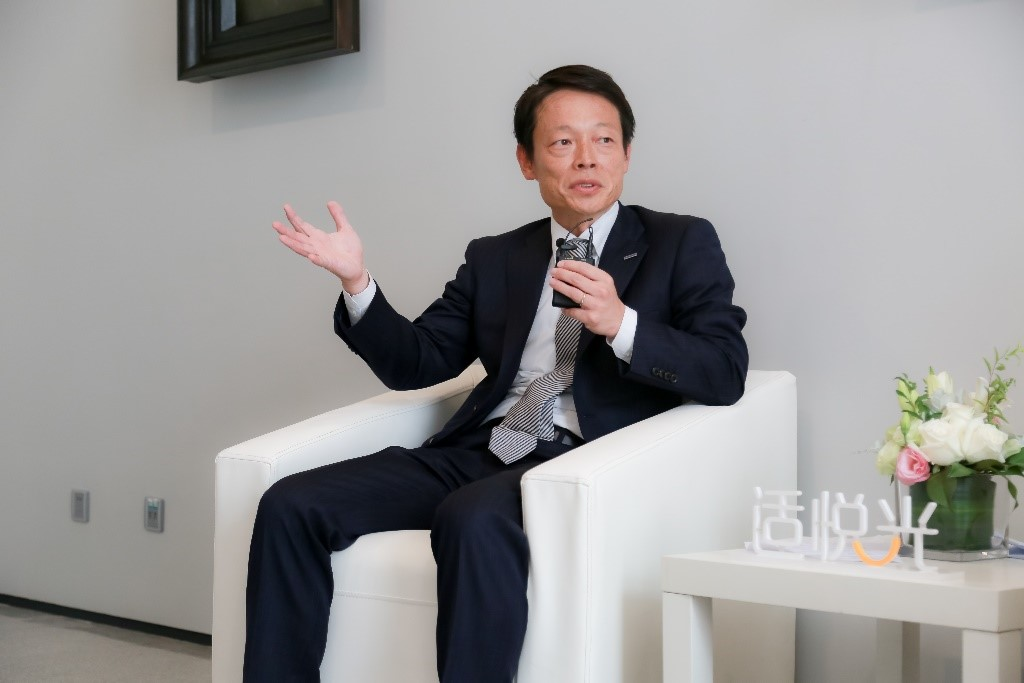“高品质是松下照明事业长久发展的基石，在此基础之上，我们将通过提供更多的产品附加价值来满足消费者更加多样化的需求，例如，推出相对应的老龄化产品、智能化产品。同时，松下照明还将着眼于更多领域的合作，拓展商品的差异化。” 少路政彦如是说。“松下一直致力于为消费者打造‘健康’、‘余暇‘、‘品味‘的家居生活空间。‘适悦光’照明新品是松下智能家居生态圈中的重要一环。我们会联合公司旗下其他事业领域，为消费者打造更加完整的智能家居生态。“

中国市场是松下全球布局中的重要一环

在被问及中国照明市场对松下照明事业的重要性时，少路政彦谈到：“中国的家居照明市场是一个蓬勃发展的市场，并且每年实现着飞速增长。与此同时，消费者自身也在不断发生着变化。松下照明将抓住市场发展机遇，沿用将中国市场定位为最重要市场的方针，致力于推动中国照明事业的进步。”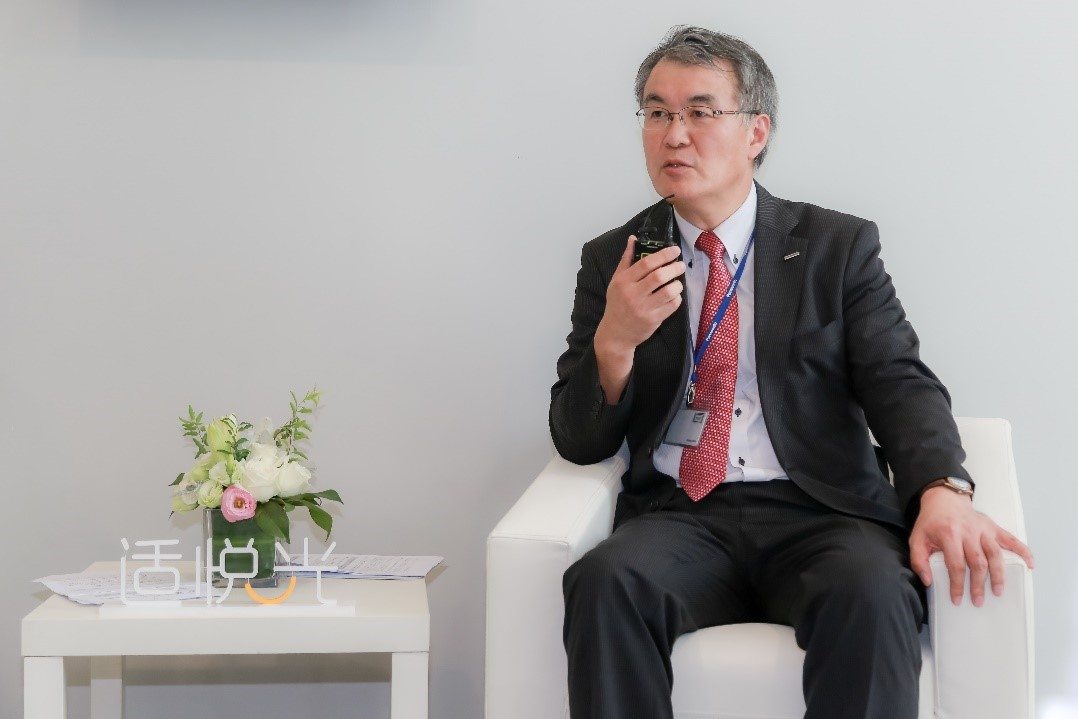少路政彦特别强调了松下照明对用户体验的重视，“松下照明有上千家线下实体店铺，能够让消费者切实感受到产品的魅力。我们将以此次“适悦光”新品上市为契机，推动现有实体店铺的革新发展，对店铺展示形象进行升级，并在主力店打造“体验情景”，让消费者不仅能够感受到松下照明产品的温度，更能体验到光的魅力。”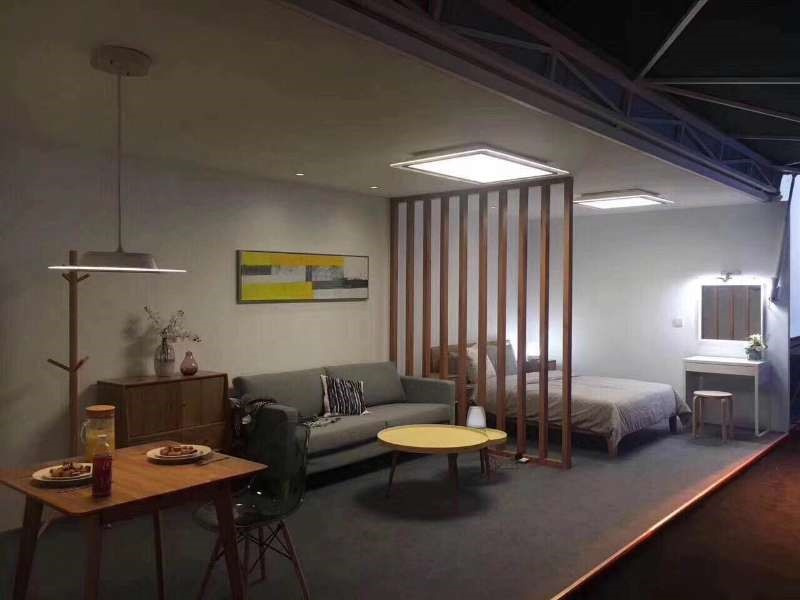松下照明秉承“A Better Life, A Better World”的美好愿景，将科技与人文融合，精心打造出“适悦光”系列照明新品，从家庭中不同居住者的角度出发，以满足家居生活中对光环境的不同需求为目的，用科技还原生活本来的简单与舒适。这一系列新品的推出，不仅为家居生活提供了极佳的照明方案，也使松下再一次在国内照明市场中树立起了全新的品质标杆。

`声明：本文由入驻焦点开放平台的作者撰写，除焦点官方账号外，观点仅代表作者本人，不代表焦点立场错误信息举报电话： 400-099-0099，邮箱：jubao@vip.sohu.com，或点此进行意见反馈，或点此进行举报投诉。`A B C D E F G H J K L M N P Q R S T W X Y Z
A - B - C - D - E
• A
• 鞍山
• 安庆
• 安阳
• 安顺
• 安康
• 澳门
• B
• 北京
• 保定
• 包头
• 巴彦淖尔
• 本溪
• 蚌埠
• 亳州
• 滨州
• 北海
• 百色
• 巴中
• 毕节
• 保山
• 宝鸡
• 白银
• 巴州
• C
• 承德
• 沧州
• 长治
• 赤峰
• 朝阳
• 长春
• 常州
• 滁州
• 池州
• 长沙
• 常德
• 郴州
• 潮州
• 崇左
• 重庆
• 成都
• 楚雄
• 昌都
• 慈溪
• 常熟
• D
• 大同
• 大连
• 丹东
• 大庆
• 东营
• 德州
• 东莞
• 德阳
• 达州
• 大理
• 德宏
• 定西
• 儋州
• 东平
• E
• 鄂尔多斯
• 鄂州
• 恩施
F - G - H - I - J
• F
• 抚顺
• 阜新
• 阜阳
• 福州
• 抚州
• 佛山
• 防城港
• G
• 赣州
• 广州
• 桂林
• 贵港
• 广元
• 广安
• 贵阳
• 固原
• H
• 邯郸
• 衡水
• 呼和浩特
• 呼伦贝尔
• 葫芦岛
• 哈尔滨
• 黑河
• 淮安
• 杭州
• 湖州
• 合肥
• 淮南
• 淮北
• 黄山
• 菏泽
• 鹤壁
• 黄石
• 黄冈
• 衡阳
• 怀化
• 惠州
• 河源
• 贺州
• 河池
• 海口
• 红河
• 汉中
• 海东
• 怀来
• I
• J
• 晋中
• 锦州
• 吉林
• 鸡西
• 佳木斯
• 嘉兴
• 金华
• 景德镇
• 九江
• 吉安
• 济南
• 济宁
• 焦作
• 荆门
• 荆州
• 江门
• 揭阳
• 金昌
• 酒泉
• 嘉峪关
K - L - M - N - P
• K
• 开封
• 昆明
• 昆山
• L
• 廊坊
• 临汾
• 辽阳
• 连云港
• 丽水
• 六安
• 龙岩
• 莱芜
• 临沂
• 聊城
• 洛阳
• 漯河
• 娄底
• 柳州
• 来宾
• 泸州
• 乐山
• 六盘水
• 丽江
• 临沧
• 拉萨
• 林芝
• 兰州
• 陇南
• M
• 牡丹江
• 马鞍山
• 茂名
• 梅州
• 绵阳
• 眉山
• N
• 南京
• 南通
• 宁波
• 南平
• 宁德
• 南昌
• 南阳
• 南宁
• 内江
• 南充
• P
• 盘锦
• 莆田
• 平顶山
• 濮阳
• 攀枝花
• 普洱
• 平凉
Q - R - S - T - W
• Q
• 秦皇岛
• 齐齐哈尔
• 衢州
• 泉州
• 青岛
• 清远
• 钦州
• 黔南
• 曲靖
• 庆阳
• R
• 日照
• 日喀则
• S
• 石家庄
• 沈阳
• 双鸭山
• 绥化
• 上海
• 苏州
• 宿迁
• 绍兴
• 宿州
• 三明
• 上饶
• 三门峡
• 商丘
• 十堰
• 随州
• 邵阳
• 韶关
• 深圳
• 汕头
• 汕尾
• 三亚
• 三沙
• 遂宁
• 山南
• 商洛
• 石嘴山
• T
• 天津
• 唐山
• 太原
• 通辽
• 铁岭
• 泰州
• 台州
• 铜陵
• 泰安
• 铜仁
• 铜川
• 天水
• 天门
• W
• 乌海
• 乌兰察布
• 无锡
• 温州
• 芜湖
• 潍坊
• 威海
• 武汉
• 梧州
• 渭南
• 武威
• 吴忠
• 乌鲁木齐
X - Y - Z
• X
• 邢台
• 徐州
• 宣城
• 厦门
• 新乡
• 许昌
• 信阳
• 襄阳
• 孝感
• 咸宁
• 湘潭
• 湘西
• 西双版纳
• 西安
• 咸阳
• 西宁
• 仙桃
• 西昌
• Y
• 运城
• 营口
• 盐城
• 扬州
• 鹰潭
• 宜春
• 烟台
• 宜昌
• 岳阳
• 益阳
• 永州
• 阳江
• 云浮
• 玉林
• 宜宾
• 雅安
• 玉溪
• 延安
• 榆林
• 银川
• Z
• 张家口
• 镇江
• 舟山
• 漳州
• 淄博
• 枣庄
• 郑州
• 周口
• 驻马店
• 株洲
• 张家界
• 珠海
• 湛江
• 肇庆
• 中山
• 自贡
• 资阳
• 遵义
• 昭通
• 张掖
• 中卫

1室1厅1厨1卫1阳台

1
2
3
4
5

0
1
2

1

1

0
1
2
3报名成功，资料已提交审核A B C D E F G H J K L M N P Q R S T W X Y Z
A - B - C - D - E
• A
• 鞍山
• 安庆
• 安阳
• 安顺
• 安康
• 澳门
• B
• 北京
• 保定
• 包头
• 巴彦淖尔
• 本溪
• 蚌埠
• 亳州
• 滨州
• 北海
• 百色
• 巴中
• 毕节
• 保山
• 宝鸡
• 白银
• 巴州
• C
• 承德
• 沧州
• 长治
• 赤峰
• 朝阳
• 长春
• 常州
• 滁州
• 池州
• 长沙
• 常德
• 郴州
• 潮州
• 崇左
• 重庆
• 成都
• 楚雄
• 昌都
• 慈溪
• 常熟
• D
• 大同
• 大连
• 丹东
• 大庆
• 东营
• 德州
• 东莞
• 德阳
• 达州
• 大理
• 德宏
• 定西
• 儋州
• 东平
• E
• 鄂尔多斯
• 鄂州
• 恩施
F - G - H - I - J
• F
• 抚顺
• 阜新
• 阜阳
• 福州
• 抚州
• 佛山
• 防城港
• G
• 赣州
• 广州
• 桂林
• 贵港
• 广元
• 广安
• 贵阳
• 固原
• H
• 邯郸
• 衡水
• 呼和浩特
• 呼伦贝尔
• 葫芦岛
• 哈尔滨
• 黑河
• 淮安
• 杭州
• 湖州
• 合肥
• 淮南
• 淮北
• 黄山
• 菏泽
• 鹤壁
• 黄石
• 黄冈
• 衡阳
• 怀化
• 惠州
• 河源
• 贺州
• 河池
• 海口
• 红河
• 汉中
• 海东
• 怀来
• I
• J
• 晋中
• 锦州
• 吉林
• 鸡西
• 佳木斯
• 嘉兴
• 金华
• 景德镇
• 九江
• 吉安
• 济南
• 济宁
• 焦作
• 荆门
• 荆州
• 江门
• 揭阳
• 金昌
• 酒泉
• 嘉峪关
K - L - M - N - P
• K
• 开封
• 昆明
• 昆山
• L
• 廊坊
• 临汾
• 辽阳
• 连云港
• 丽水
• 六安
• 龙岩
• 莱芜
• 临沂
• 聊城
• 洛阳
• 漯河
• 娄底
• 柳州
• 来宾
• 泸州
• 乐山
• 六盘水
• 丽江
• 临沧
• 拉萨
• 林芝
• 兰州
• 陇南
• M
• 牡丹江
• 马鞍山
• 茂名
• 梅州
• 绵阳
• 眉山
• N
• 南京
• 南通
• 宁波
• 南平
• 宁德
• 南昌
• 南阳
• 南宁
• 内江
• 南充
• P
• 盘锦
• 莆田
• 平顶山
• 濮阳
• 攀枝花
• 普洱
• 平凉
Q - R - S - T - W
• Q
• 秦皇岛
• 齐齐哈尔
• 衢州
• 泉州
• 青岛
• 清远
• 钦州
• 黔南
• 曲靖
• 庆阳
• R
• 日照
• 日喀则
• S
• 石家庄
• 沈阳
• 双鸭山
• 绥化
• 上海
• 苏州
• 宿迁
• 绍兴
• 宿州
• 三明
• 上饶
• 三门峡
• 商丘
• 十堰
• 随州
• 邵阳
• 韶关
• 深圳
• 汕头
• 汕尾
• 三亚
• 三沙
• 遂宁
• 山南
• 商洛
• 石嘴山
• T
• 天津
• 唐山
• 太原
• 通辽
• 铁岭
• 泰州
• 台州
• 铜陵
• 泰安
• 铜仁
• 铜川
• 天水
• 天门
• W
• 乌海
• 乌兰察布
• 无锡
• 温州
• 芜湖
• 潍坊
• 威海
• 武汉
• 梧州
• 渭南
• 武威
• 吴忠
• 乌鲁木齐
X - Y - Z
• X
• 邢台
• 徐州
• 宣城
• 厦门
• 新乡
• 许昌
• 信阳
• 襄阳
• 孝感
• 咸宁
• 湘潭
• 湘西
• 西双版纳
• 西安
• 咸阳
• 西宁
• 仙桃
• 西昌
• Y
• 运城
• 营口
• 盐城
• 扬州
• 鹰潭
• 宜春
• 烟台
• 宜昌
• 岳阳
• 益阳
• 永州
• 阳江
• 云浮
• 玉林
• 宜宾
• 雅安
• 玉溪
• 延安
• 榆林
• 银川
• Z
• 张家口
• 镇江
• 舟山
• 漳州
• 淄博
• 枣庄
• 郑州
• 周口
• 驻马店
• 株洲
• 张家界
• 珠海
• 湛江
• 肇庆
• 中山
• 自贡
• 资阳
• 遵义
• 昭通
• 张掖
• 中卫• 手机• 分享
• 设计
免费设计
• 计算器
装修计算器
• 入驻
合作入驻
• 联系
联系我们
• 置顶
返回顶部